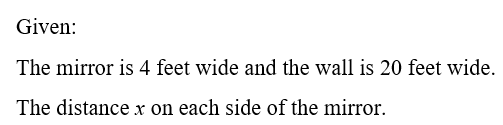# A mirror should be centered on a wall. The mirror is 4 feet wide and the wall is 20 feet wide. Which equation helps determine the distance x on each side of the mirror?x + 4 + x = 204 + x + 4 = 20x = 20 + 4 + 44 = 20 + x + x

Question
30 views

A mirror should be centered on a wall. The mirror is 4 feet wide and the wall is 20 feet wide. Which equation helps determine the distance x on each side of the mirror?
x + 4 + x = 20
4 + x + 4 = 20
x = 20 + 4 + 4
4 = 20 + x + x

check_circle

Step 1...

### Want to see the full answer?

See Solution

#### Want to see this answer and more?

Solutions are written by subject experts who are available 24/7. Questions are typically answered within 1 hour.*

See Solution
*Response times may vary by subject and question.
Tagged in